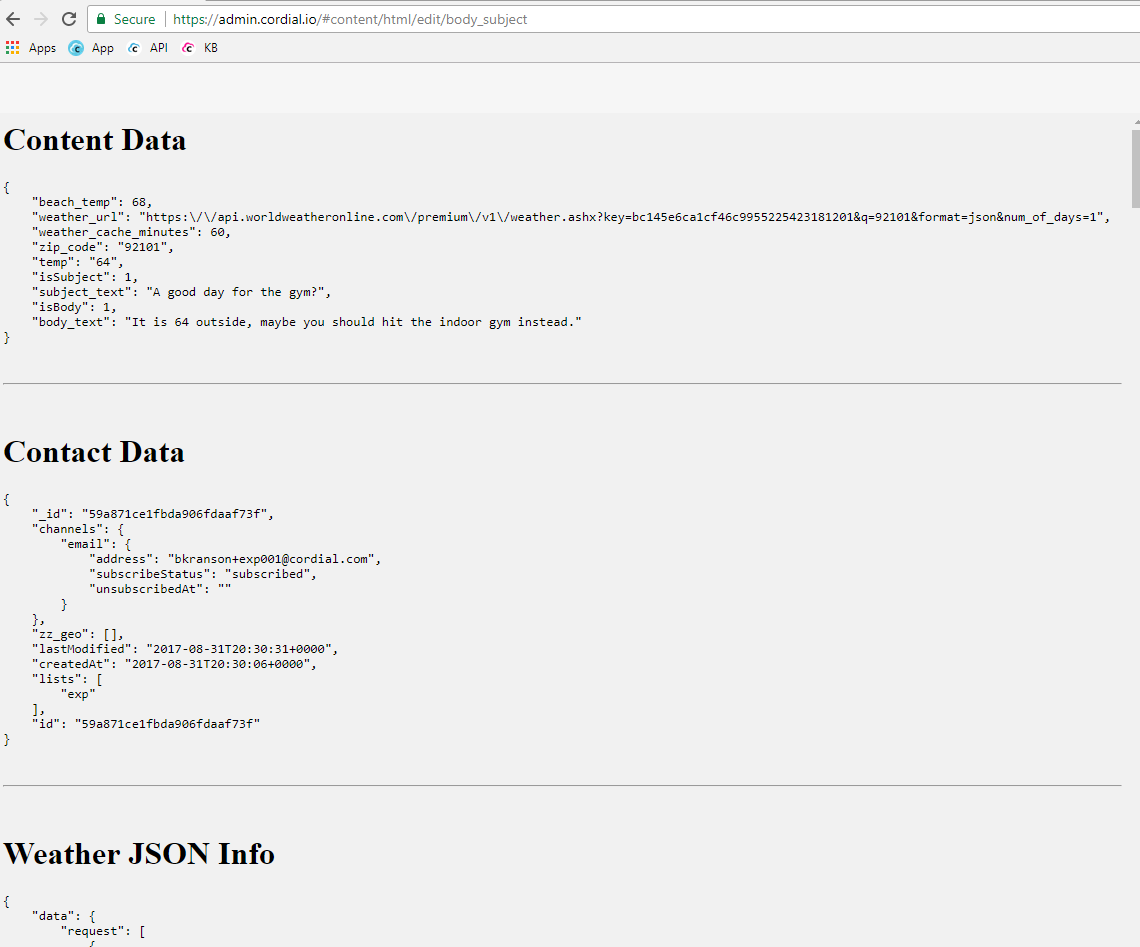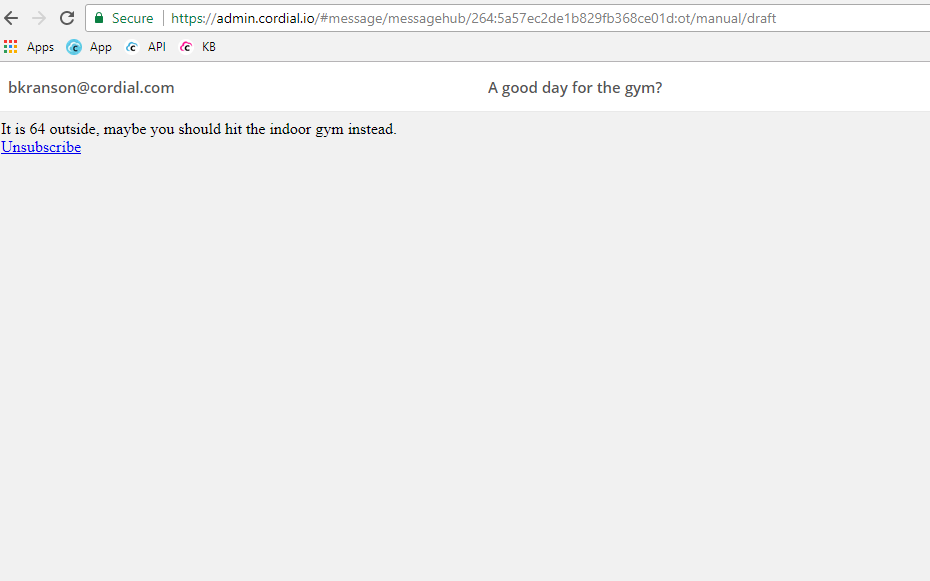# Advanced Reusable HTML Content Usage Subject

Similar to this Advanced Example, there are times where you might want to use similar logic in both the subject line and the body.  When doing complex code, we like to move the logic to an HTML Include and then use the output variables (not the HTML) in our content.

In the following example, we are going to look up the weather.  Then we are going to use the weather results in both the subject and the body.  One thing to understand here is that the code in the include will execute twice, once for subject and once for body.

To accomplish this using the Advanced method, you would start with the HTML Content.  Here is an example:

```{\$data.beach_temp = 68}
{\$data.weather_cache_minutes = 60}

{if \$contact.zip_code}
{\$data.zip_code = \$contact.zip_code}
{else}
{\$data.zip_code = '92101'}
{/if}

{\$data.weather_url = \$data.weather_url|replace:'__zip_code__':\$data.zip_code}
{\$weather=\$utils->getJson(\$data.weather_url, \$data.weather_cache_minutes)}

{if isset(\$weather) && \$weather.data.current_condition.temp_F}
{\$data.temp = \$weather.data.current_condition.temp_F}
{/if}

{* when subject and body are both not equal to 1 then we must be debugging the template *}
{if \$isSubject != 1 && \$isBody != 1}
{\$isSubject = 1}
{\$isBody = 1}
{\$debug = 1}
{/if}

{if \$isSubject == 1}
{\$data.isSubject = 1}
{\$data.subject_text = ''}
{if \$data.temp > \$data.beach_temp}
{\$data.subject_text = 'You ready for the beach?'}
{else}
{\$data.subject_text = 'A good day for the gym?'}
{/if}
{/if}

{if \$isBody == 1}
{\$data.isBody = 1}
{\$data.body_text = ''}
{if \$data.temp > \$data.beach_temp}
{\$data.body_text = "It is {\$data.temp} outside, make sure you put on some sunscreen before going to the beach."}
{else}
{\$data.body_text = "It is {\$data.temp} outside, maybe you should hit the indoor gym instead."}
{/if}
{/if}

{if \$debug == 1}
<h1>Content Data</h1>
<pre>
{\$JSON_PRETTY_PRINT = 128}
{json_encode(\$data, \$JSON_PRETTY_PRINT)}
</pre>
<br><hr><br>

<h1>Contact Data</h1>
<pre>
{\$JSON_PRETTY_PRINT = 128}
{json_encode(\$contact, \$JSON_PRETTY_PRINT)}
</pre>
<br><hr><br>

<h1>Weather JSON Info</h1>
<pre>
{\$JSON_PRETTY_PRINT = 128}
{json_encode(\$weather, \$JSON_PRETTY_PRINT)}
</pre>
<br><hr><br>
{/if}```

Now we need to use this this code in the subject.  You will notice below that we start by setting isSubject, this gets used inside the include to know which code is only to be executed for the subject line.

`{\$isSubject=1}{include 'content:body_subject' assign='blackhole' scope='parent'}{\$data.subject_text}`

In the body we set isBody similar to isSubject.

```{\$isBody=1}{include 'content:body_subject' assign='blackhole' scope='parent'}
<div>{\$data.body_text}</div>```

When you preview the HTML Content, you will get some debugging output.And when you preview the Message you can see the output of the subject and body.<% if (previousArticle || nextArticle) { %>
<% if (previousArticle) { %>
<% if (previousTitle) { %>

#### <%= previousTitle %>

<% } %> <%= previousArticle.title %>
<% } %> <% if (nextArticle) { %>
<% if (nextTitle) { %>

#### <%= nextTitle %>

<% } %> <%= nextArticle.title %>
<% } %>
<% } %>
<% if (allItems.length > 1) { %>